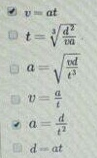# Problem: Based on these units, select any of the following expressions that could be valid expressions?

###### FREE Expert Solution

v = at = (m/s2)(s) = m/s Correct unit for v

t = (d2/va)(1/3) = (m2/((m/s)(m/s2)(1/3) = (m2/(m2/s)3)(1/3) = s Correct unit for time.

90% (35 ratings)###### Problem Details

Based on these units, select any of the following expressions that could be valid expressions?